## Simulating Matérn hard-core point processes

If you wanted to create a point process with repulsion, a reasonable first attempt would be to build off a Poisson point process by removing points according to some rule to ensure that no two points were within a certain distance of each other. Using this natural idea, Bertril Matérn proposed a family of repulsive point processes called Matérn hard-core point processes.

More specifically, Matérn proposed several points processes, including two types of hard-core point processes now called Type I and Type II. (Matérn proposed a third type, called Type III, but it’s considerably harder to simulate on a computer, as detailed in this article.) These types of hard-core point processes are completely different to the Matérn cluster point process.

As I discussed in a previous post, the Poisson point process may not be adequate for representing point phenomena whose points exhibit large degrees of repulsion or clustering. I already covered the Matérn and Thomas cluster point processes, which show distinct clustering in their configurations. In this post, I’ll cover Matérn hard-core point processes. The Type I point processes is the easier of the two, so I’ll start with that one.

## Overview

Simulating Matérn hard-core point processes requires first simulating a homogeneous Poisson point process with an intensity $$\lambda>0$$ on some simulation window, such as a rectangle, which is the simulation window I will use here. I have already written about simulating the homogeneous Poisson point processes on a rectangle and a disk, so those posts are good starting points.

Given the Poisson point process, the points then need to be thinned in such a manner to ensure that for each point, there is no other point within some fixed $$r>0$$ of the point. This distance $$r>0$$ is the radius of the hard core of each point.

I have already covered the point process operation of thinning. But it’s important to note here that in this construction a dependent thinning is being applied. (If I just applied an independent thinning, then the resulting point process will be another Poisson point process with no repulsion between points.)

## Edge effects

The main trick behind sampling this point process is that it’s possible for points inside the simulation window to be thinned due to their closeness to points that are located outside the simulation window. In other words, points outside the simulation window can cause points inside the window to be thinned. (I discussed a very similar issue in the posts on the Matérn and Thomas cluster point processes.)

To remove these edge effects, the underlying Poisson point process must be simulated on an extended version of the simulation window. The points are then thinned according to a dependent thinning, which is covered in the next section. Then only the retained points inside the simulation window are kept and the remaining points are ignored. Consequently, the underling Poisson points are simulated on an extended window, but we only see the final points inside the simulation window.

To create the extended simulation window, we add a strip of width $$r$$ all around the simulation window. Why? Well, the distance $$r$$ is the maximum distance from the simulation window that another point (outside the simulation window) can exist, while still causing points inside the simulation window to be thinned. This means it is impossible for a hypothetical point beyond this distance (outside the extended window) to cause a point inside the simulation window to be thinned.

## Dependent thinning rules

##### Type I

For each point inside the simulation window, check if there are any other points (including those in the extended window) within distance $$r$$ of the point. If no, then keep the point. If yes, then remove the point and the points that are within distance $$r$$ of the point. The remaining points inside the simulation window form a Matérn Type I point process.

This is a relatively simple thinning rule, which only requires calculating all the inter-point distances. But it is also a very strong thinning rule, meaning that it removes many points. Depending on the Poisson point process intensity $$\lambda$$ and core radius $$r$$, it is quite possible that all the points are removed, resulting in an empty configuration.

Now we examine the case when the thinning rule is not as strong.

##### Type II

To create Matérn Type II point process, we assign an independent uniform random variable to each point of the underlying Poisson point process defined on the extended window. In point process terminology, these random variables are called marks, resulting in a marked point process. In the the context of the Matérn Type II point process, these random random marks are usually called ages.

Then for each point in the simulation window, we consider all the points within distance $$r$$ of the point. If this point is the youngest (or, equivalently, the oldest) point, then the point is kept. In other words, the point is only kept if its random mark is smaller (or larger) than the random marks of all the other points within distance $$r$$ of the point. The remaining points inside the simulation window form a Matérn Type II point process.

## Intensity expressions

Using point process and probability theory, one can derive mathematical expressions for the intensities (that is, the average density of points per unit area). These closed-form expressions can then be used to check that the correct number of points are being generated on average over many simulations.

##### Type I

The intensity of the Type I point process is given by

$\mu_1=\lambda e^{-\lambda \pi r^2},$

where $$\lambda \pi r^2$$ is simply the area of the core.

##### Type II

The intensity of the Type II point process is given by

$\mu_2=\frac{1}{\pi r^2}(1-e^{-\lambda \pi r^2}),$

which can be written with the intensity of the the Type I point process as

$\mu_2=\frac{1}{\pi r^2}(1-\frac{\mu_1}{\lambda}).$

## Code

I wrote the sampling code in MATLAB and Python, which are, as usual, very similar to each other. The code, which is is located here, simulates both Type I and II Matérn points processes. It also compares the empirical intensity to the the values given by the mathematical expressions in the previous section.

##### MATLAB

The MATLAB code is here.

##### Python

The Python code is here.

## Results

I have plotted single realizations of the Matern Type I and II point processes, as well as the underlying Poisson point process in the same window.

##### MATLAB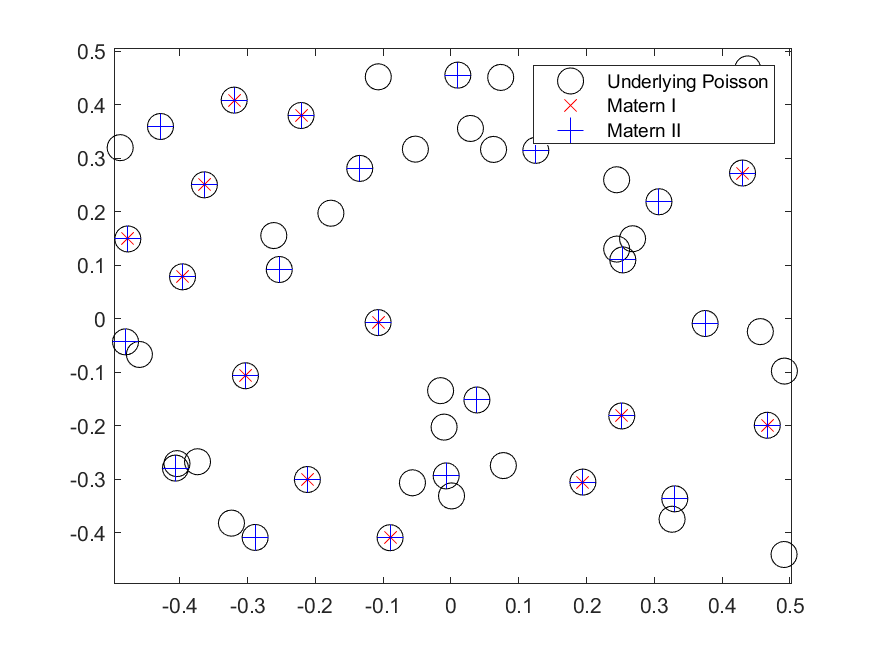##### Python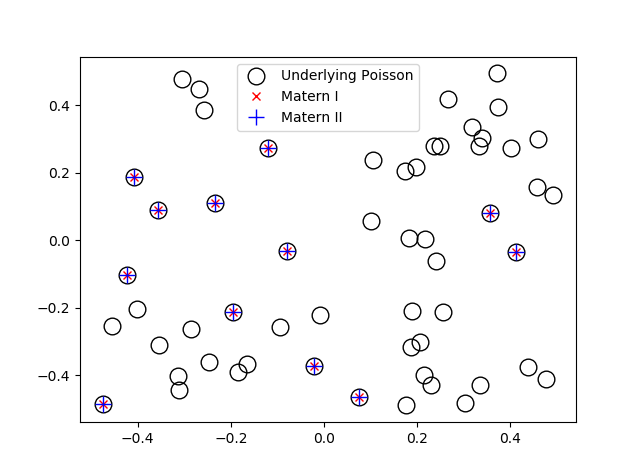Matérn hard-core point processes are covered in standard books on the related fields of spatial statistics, point processes and stochastic geometry, such as the following: Spatial Point Patterns: Methodology and Applications with R by Baddeley, Rubak and Turner, on page 140; Statistical Analysis and Modelling of Spatial Point Patterns Statistics by Illian, Penttinen, Stoyan, amd Stoyan, Section 6.5.2, starting on page 388; and; Stochastic Geometry and its Applications by Chiu, Stoyan, Kendall and Mecke, Section 5.4, starting on page 176. The first two books are particularly good for beginners.

The book Spatial Point Patterns: Methodology and Applications with R is written by spatial statistics experts Baddeley, Rubak and Turner. It covers the spatial statistics (and point process simulation) R-package spatstat., which has the functions rMaternI and rMaternII for simulating the two point processes respectively.

## Simulating Poisson random variables – Survey of methods

In the previous post, I discussed how to sample or generate Poisson random variables or (more correctly) variates. I detailed a direct method that uses the fact that a Poisson stochastic process, which is directly related to a Poisson point process, has inter-arrival times that form independent and identically distributed exponential variables. This method in turn can be easily reformulated so it only uses (standard) uniform variables to generate Poisson random variables. It is an easy and popular sampling method, explaining why it is often used.

As elegant and precise as this simulation method is, it unfortunately decreases in speed as the Poisson parameter $$\lambda$$ increases. In a tutorial published in 1983, Brian D. Ripely, a major figure in spatial statistics, says this about the direct method:

This is simple, but has expected time proportional to $$\lambda$$. Some of its competitors use rejection methods with the envelope distribution that of the integer part of a continuous random variable, such as logistic, Laplace and normal mixed with exponential distributions.

We recall that acceptance-rejection or rejections methods involve simulating a random object, such as a random variable, by first simulating another random object of the same type that is easier to simulate, and then accepting or rejecting these random variables or variates based on a certain ratio. The distribution of the random object that is first simulated is called the envelope distribution. Such rejection methods are one way to simulate Poisson variables.

Consequently, the code of most computer functions for generating Poisson variables will have an if-statement, using the direct method for small parameter values and another method for large parameter values.

### Different methods

Over the years there have been different methods proposed for producing Poisson random variates. In the book Non-uniform random variate generation, Luc Devroye groups the different methods into five categories with his views, which I’ll briefly describe here:

1. Direct methods based on the homogeneous Poisson stochastic process having exponential inter-arrival times. These methods are simple, but the expected time is proportional to the Poisson parameter $$\lambda$$.
2. Inversion methods that search through a table of cumulative Poisson probabilities. Examples include the papers by Fishman (1976) and Atkinson (1979)*.
3. Methods that use the recursive properties of the Poisson distribution. The paper by Ahrens and Dieter (1974) uses this approach, and its expected time of completion is proportional to $$\log\lambda$$.
4. Acceptance-rejection (or rejection) methods that give relatively fast but simple algorithms. Such methods are proposed in the papers by Atkinson (1979)*, Ahrens and Dieter (1980) and Devroye (1981) or the technical report by Schmeiser and Kachitvichyanukul (1981).
5. Acceptance-complement methods that uses a normal distribution as the starting distribution, such as the paper by Ahrens and Dieter (1982). This method is fast, but the code is rather long.

*Atkinson had (at least) two papers on generating Poisson variates published in 1979, but I believe Devroye is referring to the first paper, because in the second paper Atkinson compares methods proposed by others.

For titles of the papers, see the Further reading section below.

## Methods implemented

In this section, I’ll state which proposed methods are used in various programming languages and numerical methods. I won’t go into the details how the methods work, as I’ll just cite the papers instead.

##### MATLAB

For small $$\lambda$$ values, the MATLAB function poissrnd uses the  direct method (based on inter-arrival times) with a while-loop.

For $$\lambda$$ values greater than fifteen, I believe that the MATLAB function poissrnd uses Algorithm PG from the 1974 paper by Ahrens and Dieter. But to come to this conclusion, I had to do some investigating. You can skip to the next section if you’re not interested, but now I’ll explain my reasoning.

The MATLAB documentation says it uses a method proposed by Ahrens and Dieter, but these two researchers developed a number of methods for generating Poisson variables. The MATLAB code cites Volume 2 of the classic series by Knuth, who says the method is due to Ahrens and Dieter, but he doesn’t give an exact citation in that section of the book. Confusingly, Knuth cites in his book a couple papers by Ahrens and Dieter for generating different random variates. (Knuth later cites a seemingly relevant 1980 paper by Ahrens and Dieter, but that details another method.)

Both the MATLAB code and Knuth cite the book by Devroye. In his book (Exercise 3.5.2), Devroye discusses one method, among others, from a 1974 paper by Ahrens and Dieter. Another hint is given by examining the code of the MATLAB function poissrnd, which reveals that it uses the function randg to generate gamma variables. In the Ahrens and Dieter 1974 paper, their Algorithm PG (for producing Poisson variates) uses gamma random variables, and it’s suggested to use a parameter value of $$7/8$$, as used by MATLAB and mentioned by Knuth, confirming this is the right paper.

In summary, for large $$\lambda$$ the function MATLAB uses Algorithm PG from the 1974 paper by Ahrens and Dieter, whereas it uses the direct method for small values.

##### R

In R, the function rpois use an algorithm outlined in the 1982 paper by Ahrens and Dieter. You can view the R source code here. The two cases for $$\lambda$$ (or $$\mu$$ in the paper) depend on whether $$\lambda$$ is greater than ten or not. For small $$\lambda$$, the R function rpois does not use the method based on inter-arrival times, but rather an inversion method based on a table of (cumulative) probabilities given by the Poisson probability distribution.

##### Python (NumPy)

In NumPy, the function numpy.random.poisson generates Poisson variates. The source code for the NumPy library is here, but for Poisson function the underlying code is actually written in C; see the distributions.c file located here. For small Poisson parameter $$\lambda$$, the code uses the direct method; see the function random_poisson_mult in the code.

For Poisson parameter $$\lambda \geq 10$$, the comments in the code reveal that it uses a method from a 1993 paper by Hörmann; see Algorithm PTRS on page 43 of the paper. This is a transformation method, which for NumPy is implemented in the C code as the function random_poisson_ptrs. The method, which Hörmann calls the transformed rejection with squeeze, combines inversion and rejection methods.

##### Octave

Octave is intended to be a GNU clone of MATLAB, so you would suspect it uses the same methods as MATLAB for generating Poisson random variates. But the Octave function poissrnd uses different methods. The code reveals it generates the Poisson variates with a function called prand. It considers different cases depending on the value of the Poisson parameter $$\lambda$$ as well as whether a single variable (that is, a scalar) or vector or matrix of Poisson variates are being generated.

In total, the Octave function prand uses five different methods. For two of the methods, the documentation cites methods from the classic book Numerical Recipes in C (the 1992 edition); see next section. To generate a single Poisson variate with Poisson parameter $$\lambda \leq 12$$, the Octave function prand uses the direct method based on inter-arrival times.

##### Numerical Recipes (Fortran, C and C++)

The Numerical Recipes is a classic book by Press, Teukolsky, Vetterling and Flannery on numerical methods. The books comes in different editions reflecting different publication years and computer languages. (In the first two editions of the book, the authors implemented the algorithms respectively in Fortran and C.)

For generating Poisson variates, the book contents seems to have not changed over the editions that I looked at, which covered the programming languages Fortran (77 and 90), C, and C++. The authors cover Poisson generation in Section 7.3 in the Fortran and C editions. In the third edition of Numerical Recipes, they implement their methods in C++ in Section 7.3.12.

For small values of Poisson parameter $$\lambda$$, Numerical Recipes uses the direct method. For $$\lambda >12$$ values, an acceptance-rejection method is used, which relies upon finding a continuous version of the discrete Poisson probability distribution.

##### GSL Library (C)

In the GSL library, one can use the function gsl_ran_poisson, which uses the the direct method of exponential times. The code, which can be viewed here, cites simply Knuth (presumably the second volume).

##### NAG Library (C)

Although I didn’t see the code, it appears that the function nag_rand_poisson (g05tjc ) in the NAG library also uses the direct method, based on the material in the second volume of series by Knuth. But in a 1979 paper Atkinson says that the NAG library uses a method from the 1974 paper by Ahrens and Dieter.

##### MKL library (C)

In the MKL C library written by Intel, they seem to have three functions or methods for generating Poisson variates.

The first function is called VSL_RNG_METHOD_POISSON_PTPE, which does the following for a Poisson distribution with parameter $$\Lambda$$:

If Λ ≥ 27, random numbers are generated by PTPE method. Otherwise, a combination of inverse transformation and table lookup methods is used. The PTPE method is a variation of the acceptance/rejection method that uses linear (on the fraction close to the distribution mode) and exponential (at the distribution tails) functions as majorizing functions. To avoid time-consuming acceptance/rejection checks, areas with zero probability of rejection are introduced and a squeezing technique is applied.

This function uses the so-called PTPE method, which is outlined in a 1981 technical report by Schmeiser and Kachitvichyanukul.

The second function is called VSL_RNG_METHOD_POISSON_POISNORM, which does the following :

If Λ < 1, the random numbers are generated by combination of inverse transformation and table lookup methods. Otherwise, they are produced through transformation of the normally distributed random numbers.

The third function is called VSL_RNG_METHOD_POISSONV_POISNORM, which does the following:

If Λ < 0.0625, the random numbers are generated by inverse transformation method. Otherwise, they are produced through transformation of normally distributed random numbers.

## cuRAND (C)

Finally, Nvidia’s CUDA Random Number Generation library (cuRAND) has a function for generating Poisson variates. To see the C code, copies of it can be found in various GitHub repositories, such as this one. The cuRAND function curand_poisson uses the direct function for Poisson parameter values  less than 64. For parameters values greater than 4000, it uses a normal approximation (rounded to the nearest integer).

For other values, the function curand_poisson uses an a rejection method based on an approximation of the incomplete gamma function; see the function curand_poisson_gammainc. The book by Fishman is cited; see Section 8.16.

For various Poisson simulation methods, see the stochastic simulation books by Devroye (Section X.3) or Fishman (Section 8.16). The book by Gentle (Section 5.2.8) also briefly covers Poisson variables. Kuhl wrote a paper on the history of generating random variates. It has some interesting historical points, but I was not entirely impressed by the quality or detail of the paper.

Of course, it’s a good idea to look at the citations that the different functions use.

Finally, here is a list of the papers I mentioned in this post:

• 1974, Ahrens and Dieter, Computer methods for sampling from gamma, beta, poisson and bionomial distributions;
• 1976, Fishman, Sampling from the Poisson distribution on a computer;
• 1979, Atkinson, The computer generation of Poisson random variables;
• 1979, Atkinson, Recent developments in the computer generation of Poisson random variables;
• 1980, Ahrens and Dieter, Sampling from binomial and Poisson distributions: a method with bounded computation times;
• 1980, Devroye, The Computer Generation of Poisson Random Variables;
• 1981, Schmeiser and Kachitvichyanukul, Poisson Random Variate Generation;
• 1982, Ahrens and Dieter, Computer generation of Poisson deviates from modified normal distributions;
• 1983, Ripley, Computer Generation of Random Variables: A Tutorial;
• 1993, Hörmann, The transformed rejection method for generating Poisson random variable.

## Simulating Poisson random variables – Direct method

If you were to write from scratch a program that simulates a homogeneous Poisson point process, the trickiest part would be the random number of points, which requires simulating a Poisson random variable. In previous posts, here and here, I’ve simply used the inbuilt functions for simulating (or generating) Poisson random variables (or variates). (In the literature, researchers describe methods on how to generate random deviates or variates. But, in my informal way, I will often say simulate random variables or generate random variates, somewhat interchangeably.)

But how would one create such a function using just a standard uniform random variate generator?

The generator or method being used depends on the value of the Poisson parameter, denoted here by $$\lambda$$, which is the mean (as well as the variance) of a random variable with a Poisson distribution. If this parameter value is small, which in practice has been less than some number between 10 to 30, then a direct simulation method can be used to generate Poisson random variates.

For large $$\lambda$$ values, other methods are generally used, such as rejection or (highly accurate) approximation methods. In the book Non-uniform random variate generation, the author Luc Devroye groups the methods into five categories (Section X.3.2), which I briefly describe in the next post. The first of these categories covers the method that I mentioned above, which is what I’ll cover in this post, with some code in C and C#. (I also present some code in MATLAB, but you would never use it instead of the the inbuilt function poissrnd.)

In the next post, I’ll describe other types of Poisson simulation methods, and I’ll detail which simulation methods various programming libraries use.

### Direct method

An elegant and natural method for simulating Poisson variates is using a result based on the homogeneous Poisson stochastic process. The points in time when a given homogeneous Poisson stochastic process increases forms a Poisson point process on the real line. Confusingly, the term Poisson process is often used to refer to both the stochastic process and the point process, but there is a slight difference.

##### Using exponential random variables

The times when the Poisson stochastic process increases are called arrival times or occurrence times, as in classic stochastic models they represent the arrivals or occurrences of something, such as phone calls over time. The differences between consecutive times are called inter-arrival times or inter-occurrence times. The inter-arrival times of a homogeneous Poisson process form independent exponential random variables, a result known as the Interval Theorem.

Using this connection to the Poisson stochastic process, we can generate exponential variables $$E_1$$, $$E_2, \dots$$, and add them up. The smallest number of exponential variables for the resulting sum to exceeds one will give a Poisson random variable. That is, if we define $$N$$ to be the smallest $$n$$ such that
$$\sum_{k=1}^n E_k > 1,$$
then $$N$$ is a random variable distributed according to a Poisson distribution.

Generating exponential variates is easily done by using the inverse method. For a uniform random variable $$U$$ on the unit interval $$(0,1)$$, the transformation $$E= -\log(U)/\lambda$$ gives an exponential random variable with mean $$1/\lambda$$.

##### Using uniform random variables

To reduce computation overhead, the direct method using exponential random variables is often reformulated as products of uniform random variables, due to logarithmic identities, instead of sums of exponential random variables. Then using standard uniform random variables $$U_1, U_2,\dots$$, we define $$N$$ to be the smallest $$n$$ such that
$$\prod_{k=1}^n U_k > e^{-\lambda}.$$

These two different formulations of the same method are captured by Lemma 3.2 and Lemma 3.3 in Chapter 10 of Devroye’s book.

##### Example in MATLAB

In MATLAB, we can implement this method with the first formulation in a function with a simple while-loop:

function N=funPoissonLoop(lambda)
T=0; %initialize sum of exponential variables as zero
n=-1;%initialize counting variable as negative one

while (T < 1)
E=-(1/lambda)*log(rand(1));%generate exponential random variable
T=T+E; %update sum of exponential variables
n=n+1; %update number of exponential variables
end
N=n;
end


But, as I said before, don’t use this code instead of the inbuilt function poissrnd.

If you want to be a bit more tricky, you could achieve the same result by using recursion:

function N=funPoissonRecursive(lambda)
T=0; %initialize sum of exponential variables as zero
n=-1; %initialize counting variable as negative one

%run (recursive) exponential function step function
[~,N]=funStepExp(lambda,T,n);

function [T,N]=funStepExp(nu,S,m)
if (S < 1)
%run if sum of exponential variables is not high enough

%generate exponential random variable
E=(-log(rand(1)))/nu;
S=S+E; %update sum of exponential variables
m=m+1; %update nunber of exponential variables

%recursively call function again
[T,N]=funStepExp(nu,S,m);
else
T=S;
N=m;
end
end
end


Note how the code recursively calls the function funStepExp, which generates an exponential variable each time.

In the Code section below I describe my code in C and C#, using the second formulation.

### Origins

Some people attribute the direct method, based on inter-arrival times, to (or, at least, cite) Donald Knuth, who details it in the second volume of his classic series of books, but I doubt that the great Knuth was the first to have this idea. For example, a quick search on Google Scholar found a paper  by K. D. Tocher on computers and random sampling, where Tocher proposes the direct method in 1954, some years before Knuth started publishing his classic series.

The direct method for Poisson sampling relies upon the Interval theorem. Günter Last studied its history  in a recent book authored by him and Matthew Penrose; see Chapter 7 and its corresponding historical footnotes in Section C of the appendix. (A free version of the book can be found here. ) People connected to the result include Robert Ellis and William Feller.

### Other methods

The direct method perfectly generates Poisson random variables (or I should say Poisson random variates). But it can be too slow for large values of the Poisson parameter (that, is the mean) $$\lambda$$. This has motivated researchers to develop other methods, which I will mention in the next post.

### Code

I wrote some code that simulates Poisson random variables by employing the direct method based on exponential inter-arrival times. I have implemented the second formulation (using just uniform variables) in the C and C# languages. In the code, I have used a while-loop to implement the method, but I could have also used a recursion method, as I did in the MATLAB example above, which I have also done in Python (with NumPy).

For an empirical test, the code also calculates the mean and variance of a collection of Poisson variables. For a large enough number of variables, the sample mean and the variance will closely agree with each other, converging to the same value.

##### C

Warning: My C code uses rand(), the standard pseudo-random number function in C, which is known for failing certain tests of randomness. The function is adequate for regular simulations. Replace this function with another pseudo-random number generator.

The code for generating a single Poisson variate is fairly straightforward in C. Here’s a sample of the code with just the Poisson function:

//Poisson function -- returns a single Poisson random variable
int funPoissonSingle(double lambda)
{
double exp_lambda = exp(-lambda); //constant for terminating loop
double randUni; //uniform variable
double prodUni; //product of uniform variables
int randPoisson = -1; //Poisson variable

//initialize variables
randPoisson = -1;
prodUni = 1;
do
{
randUni = funUniformSingle(); //generate uniform variable
prodUni = prodUni * randUni; //update product
randPoisson++; //increase Poisson variable

} while (prodUni > exp_lambda);
return randPoisson;
}


For generating multiple variates, the code becomes more complicated, as one needs to use pointers, due to the limited or static memory capabilities of C. Again, the function uses the pseudo-random number generator in C.

##### C#

The code for generating a single Poisson variate is also straightforward in C#. Here’s the function in C#:

//Poisson function -- returns a single Poisson random variable
public int funPoissonSingle (double lambda) {
double exp_lambda = Math.Exp (-lambda); //constant for terminating loop
double randUni; //uniform variable
double prodUni; //product of uniform variables
int randPoisson = -1; //Poisson variable

//initialize variables
randPoisson = -1;
prodUni = 1;
do {
randUni = funUniformSingle (); //generate uniform variable
prodUni = prodUni * randUni; //update product
randPoisson++; // increase Poisson variable

} while (prodUni > exp_lambda);

return randPoisson;
}


Generalizing the code so it generates multiple variates just requires little change, compared to C, as the C# language is a much more modern language.

For various Poisson simulation methods, see the stochastic simulation books by Devroye (Section X.3) or Fishman (Section 8.16). There’s a free online version of Devroye’s book here. The book by Gentle (Section 5.2.8) also briefly covers Poisson variables.

In this post on generating Poisson variates, John D. Cook briefly discusses the direct method for small $$\lambda$$ values and a rejection method from a 1979 paper by Atkinson, which I mention in the next post. He presents his C# sharp code in this post.

## Simulating Poisson point processes faster

As an experiment, I tried to write code for simulating many realizations of a homogeneous Poisson point process in a very fast fashion. My idea was to simulate all the realizations in two short steps.

In reality, the findings of this experiment and the contents of this post have little practical value, as computers are so fast at generating Poisson point processes. Still, it was an interesting task, which taught me a couple of things. And I did produce faster code.

### MATLAB

I first tried this experiment in MATLAB.

##### Vectorization

In languages like MATLAB, the trick for speed is to use vectorization, which means applying a single operation to an entire vector or matrix (or array) without using an explicit for-loop. Over the years, the people behind MATLAB has advised to use vectorization instead of for-loops, as for-loops considerably slowed down MATLAB code. (But, as as time goes by, it seems using for-loops in MATLAB doesn’t slow the code down as much as it used to.)

Simulating Poisson point processes is particularly amenable to vectorization, due to the independent nature of the point process. One can simulate the number of points in each realization for all realizations in one step. Then all the points across all realizations can also be positioned in one step. In the two-dimensional case, this results in two one-dimensional arrays (or vectors, in MATLAB parlance) for the $$x$$ and $$y$$ coordinates.  (Of course, in my code, I could have used just one two-dimensional array/vector  for the coordinates of the points, but I didn’t.)

After generating the points, the coordinates of the points need to be grouped into the different realizations and stored in appropriate data structures.

##### Data structures

The problem with storing point processes is that usually each realization has a different number of points, so more sophisticated data structures than regular arrays are needed. For MATLAB, each point process realization can be stored in a data object called a cell array.  For a structure array, it’s possible for each element (that is, structure) to be a different size, making them ideal for storing objects like point processes with randomly varying sizes.

In the case of two-dimensional point processes, two cell arrays  can be used to store the $$x$$ and $$y$$ coordinates of all the point process realizations. After randomly positioning all the points, they can be grouped into a cell array, where each cell array element represents a realization of the Poisson point process, by using the inbuilt function MATLAB mat2cell, which converts a matrix (or array) into a cell array.

Alternatively, we could use another MATLAB data object called a structure array. In MATLAB structures have fields, which can be, for example for a point process can be the locations of the points. Given cell arrays of equal size, we can convert them into a single structure array by using the inbuilt MATLAB function struct.

### Python

After successfully simulating Poisson point processes in MATLAB, I tried it in Python with the NumPy library.

##### Vectorization

I basically replicated what I did in MATLB using Python by positioning all the points in a single step. This gives two one-dimensional NumPy arrays for the $$x$$ and $$y$$ coordinates of all the point process realizations. (Again, I could have instead stored the coordinates as a single two-dimensional array, but I didn’t.)

Perhaps surprisingly, the vectorization step doesn’t speed things up much in Python with the NumPy library. This may be due to the fact that the underlying code is actually written in the C language.  That motivated me to see what methods have been implemented for simulating Poisson variables, which is the topic of the next couple posts.

##### Data structures

In Python, the data structure  known as a list is the natural choice. Similarly to cell arrays in MATLAB, two lists can be used for the $$x$$ and $$y$$ coordinates of all the points. Instead of MATLAB’s function mat2cell, I used the NumPy function numpy.split to create two lists from the two NumPy arrays containing the point coordinates.

Python does not seem to have an immediate equivalent to structure arrays in MATLAB. But in Python one can define a new data structure or class with fields, like a structure. Then one can create a list of those data structures with fields, which are called attribute references in Python.

### Code

The code in MATLAB and Python can be found here. For a comparison, I also generated the same number of point process realizations (without using vectorization) by using a trusty for-loop. The code compares the times of taken for implemented the two different approaches, labelled internally as Method A and Method B. There is a some time difference in the MATLAB code, but not much of a difference in the Python case.

I have commented out the sections that create data structures (with fields or attribute references) for storing all the point process realizations, but those sections should also work when uncommented.

## Placing a random point uniformly in a Voronoi cell

In the previous post, I discussed how Voronoi or Dirichlet tesselations are useful and how they can be calculated or estimated with many scientific programming languages by using standard libraries usually based on Qhull. The cells of such a tessellation divide the underlying space. Now I want to randomly place a single point in a uniform manner in each bounded Voronoi cell.

But why?

Well, this task arises occasionally, particularly when developing mathematical models of wireless networks, such as mobile or cellular phone networks. A former colleague of mine had to do this, which inspired me to write some MATLAB code a couple of years ago. And I’ve seen the question posed a couple of times on the web . So I thought: I can do that.

## Overview

For this problem, I see two obvious methods.

##### Simple but crude

The simplest method is to cover each Voronoi cell with a rectangle or disk. Then randomly place a point uniformly on the rectangle or disk. If it doesn’t randomly land inside the rectangle or disk, then do it again until it does. Crude, slightly inefficient, but simple.

##### Elegant but slightly tricky

A more elegant way, which I will implement, is to partition (or divide) each Voronoi cell into triangles. Then randomly choose a triangle based on its area and, finally, uniformly position a point on that triangle.

## Method

##### Partition cells into triangles

It is straightforward to divide a Voronoi cell into triangles. Each side of the cell corresponds to one side of a triangle (that is, two points). The third point of the triangle is the original point corresponding to the Voronoi cell.

##### Choose a triangle

Randomly choosing a triangle is also easy. For a given cell, number the triangles. Which random triangle is chosen is simply a discrete random variable whose probability mass function is formed from triangle areas normalized (or divided) by the total area of the Voronoi cell. In other words, the probability of randomly choosing triangle $$i$$ with area $$A_i$$ from $$m$$ triangles is simply

$$P_i=\frac{A_i}{\sum_{j=1}^m A_j}.$$

To calculate the area of the triangles, I use the shoelace formula , which for a triangle with corners labelled $$\textbf{A}$$, $$\textbf{B}$$ and $$\textbf{C}$$ gives

$$A= \frac{1}{2} |(x_{\textbf{B}}-x_{\textbf{A}})(y_{\textbf{C}}-y_{\textbf{A}})-(x_{\textbf{C}}-x_{\textbf{A}})(y_{\textbf{B}}-y_{\textbf{A}})|.$$

But you can also use Herron’s formula.

Then the random variable is sampled using the probabilities based on the triangle areas.

##### Place point on chosen triangle

Given a triangle, the final step is also easy, if you know how, which is often the case in mathematics. I have already covered this step in a previous post, but I’ll give some details here.

To position a single point uniformly in the triangle, generate two random uniform variables on the unit interval $$(0,1)$$, say $$U$$ and $$V$$. The random $$X$$ and $$Y$$ coordinates of the single point are given by the expressions:

$$X=\sqrt{U} x_{\textbf{A}}+\sqrt{U}(1-V x_{\textbf{B}})+\sqrt{U}V x_{\textbf{C}}$$

$$Y=\sqrt{U} y_{\textbf{A}}+\sqrt{U}(1-V y_{\textbf{B}})+\sqrt{U}V y_{\textbf{C}}$$

## Results

The blue points are the points of the underlying point pattern that was used to generate the Voronoi tesselation. (These points have also been arbitrarily numbered.) The red points are the random points that have been uniformly placed in all the bounded Voronoi cells.

##### MATLAB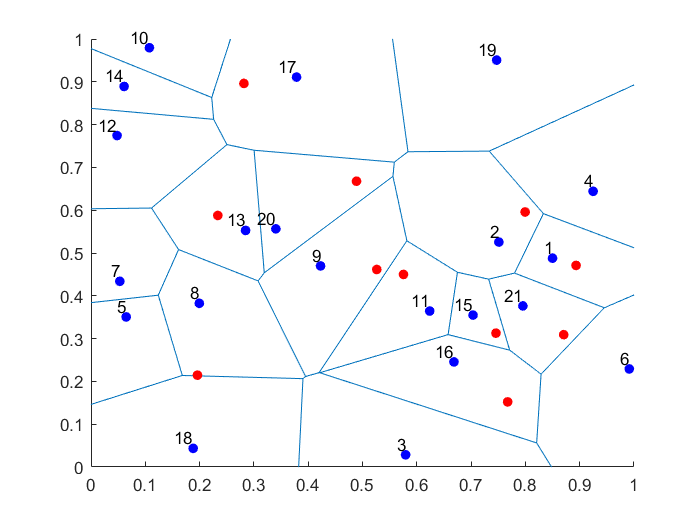##### Python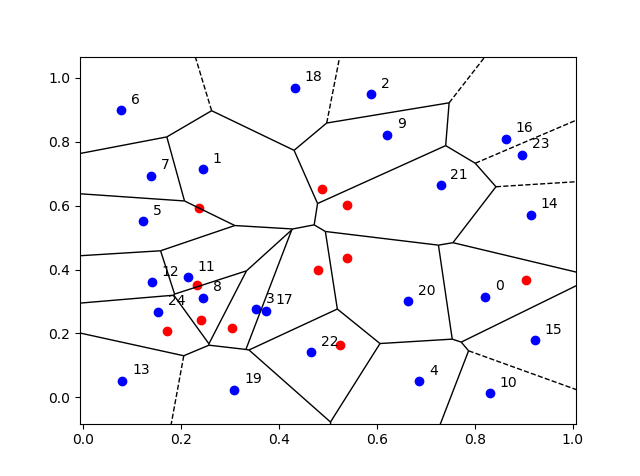## Empirical validation

We can empirically validate that the points are being placed uniformly on the bounded Voronoi cells. For a given (that is, non-random) Voronoi cell, we can repeatedly place (or sample) a random point uniformly in the cell. Increasing the number of randomly placed points, the respective averages of the $$x$$ and $$y$$ coordinates of the points will converge to the centroids (or geometric centres) of the Voronoi cell, which can be calculated with simple formulas.

## Code

The code for all my posts is located online here. For this post, the code in MATLAB and Python is here.

I have also written in MATLAB and Python the code as functions (in files funVoronoiUniform.m and funVoronoiUniform.py, respectively), so people can use it more easily. The function files are located here, where I have also included an implementation of the aforementioned empirical test involving centroids. You should be able to use those functions and for any two-dimensional point patterns.

Much has been written on Voronoi or Dirichlet tessellations, particularly when the seeds of the cells form a Poisson point process. For references, I recommend my previous post, where I say that the references in the articles on Wikipedia and MathWorld are good starting points.

In this StackExchange post, people discuss how to place a single point uniformly on a general triangle. For the formulas, the thread cites the paper Shape distributions by Osada, Funkhouser, Chazelle and Dobkin, where no proof is given.

## Simulating a Cox point process based on a Poisson line process

In the previous post, I described how to simulate a Poisson line process, which in turn was done by using insight from an earlier post on the Bertrand paradox.

Now, given a Poisson line process, for each line, if we generate an independent one-dimensional Poisson point point process on each line, then we obtain an example of a Cox point process. Cox point processes are also known as doubly stochastic Poisson point processes. On the topic of names, Guttorp and Thorarinsdottir argue that it should be called the Quenouille point process, as Maurice Quenouille introduced an example of it before Sir David Cox, but I opt for the more common name.

## Cox point proceesses

A Cox point process is a generalization of a Poisson point process. It is created by first considering a non-negative random measure, sometimes called a driving measure. Then a Poisson point process, which is independent of the random driving measure, is generated by using the random measure as its intensity or mean measure.

The driving measure of a Cox point process can be, for example, a non-negative random variable or field multiplied by a Lebesgue measure. In our case, the random measure is the underlying Poisson line process coupled with the Lebesgue measure on the line (that is, length).

Cox processes form a very large and general family of point processes, which exhibit clustering. In previous posts, I have covered two special cases of Cox point processes: the Matérn and Thomas cluster point processes. These are, more specifically, examples of a Neyman-Scott point process, which is a special case of a shot noise Cox point process. These two point processes are fairly easy to simulate, but that’s not the case for Cox point processes in general. Some are considerably easier than others.

## Motivation

I will focus on simulating the Cox point process formed from a Poisson line process with homogeneous Poisson point processes. I do this for two reasons. First, it’s easy to simulate, given we can simulate a Poisson line process. Second, it has been used and studied recently in the mathematics and engineering literature for investigating wireless communication networks in cities, where the streets correspond to Poisson lines; for example, see these two preprints:

Incidentally, I don’t know what to call this particular Cox point process. A Cox line-point process? A Cox-Poisson line-point process? But it doesn’t matter for simulation purposes.

## Method

We will simulate the Cox (-Poisson line-) point process on a disk. Why a disk? I suggest reading the previous posts on the Poisson line process the Bertrand paradox for why the disk is a natural simulation window for line processes.

Provided we can simulate a Poisson line process, the simulation method is quite straightforward, as I have essentially already described it.

##### Line process

First simulate a Poisson line process on a disk. We recall that for each line of the line process, we need to generate two independent random variables $$\Theta$$ and $$P$$ describing the position of the line. The first random variable $$\Theta$$ gives the line orientation, and it is a uniform random variable on the interval $$(0,2\pi)$$.

The second random variables $$P$$ gives the distance from the origin to the disk edge, and it is a uniform random variable on the interval $$(0,r)$$, where $$r$$ is the radius of the disk. The distance from the point $$(\Theta, P)$$ to the disk edge (that is, the circle) along the chord is:

$$Q=\sqrt{r^2-P^2}.$$

The endpoints of the chord (that is, the points on the disk edge) are then:

Point 1: $$X_1=P \cos \Theta+ Q\sin \Theta$$, $$Y_1= P \sin \Theta- Q\cos \Theta$$,

Point 2: $$X_2=P \cos \Theta- Q\sin \Theta$$, $$Y_2= P \sin \Theta+Q \cos \Theta$$.

The length of the line segment is $$2 Q$$. We can say this random line is described by the point $$(\Theta,P)$$.

##### One-dimensional Poisson point process

For each line (segment) in the line process, simulate a one-dimensional Poisson point process on it. Although I have never discussed how to simulate a one-dimensional (homogeneous) Poisson point process, it’s essentially one dimension less than simulating a homogeneous Poisson point process on a rectangle.

More specifically, given a line segment $$(\Theta,P)=(\theta,p)$$, you simulate a homogeneous Poisson point process with intensity $$\mu$$ on a line segment with length $$2 q$$, where $$q=\sqrt{r^2-p^2}$$. (I am now using lowercase letters to stress that the line is no longer random.) To simulate the homogeneous Poisson point process, you generate a Poisson random variable with parameter $$2 \mu q$$.

Now you need to place the points uniformly on the line segment. To do this, consider a single point on a single line. For this point, generate a single uniform variable $$U$$ on the interval $$(-1,1)$$. The tricky part is now getting the Cartesian coordinates right. But the above expressions for the endpoints suggest that the single random point has the Cartesian coordinates:

$$x=p \cos \theta+ U q\sin \theta$$, $$y=p \sin \theta- U q\cos \theta$$.

The two extreme cases of the uniform random variable $$U$$ (that is, $$U=-1$$ and $$U=1$$) correspond to the two endpoints of the line segment. We recall that $$Q$$ is the distance from the midpoint of the line segment to the disk edge along the line segment, so it makes sense that we want to vary this distance uniformly in order to uniformly place a point on the line segment. This uniform placement step is done for all the points of the homogeneous Point process on that line segment.

You repeat this procedure for every line segment. And that’s it: a Cox point process built upon a Poisson line process.

## Results

##### MATLAB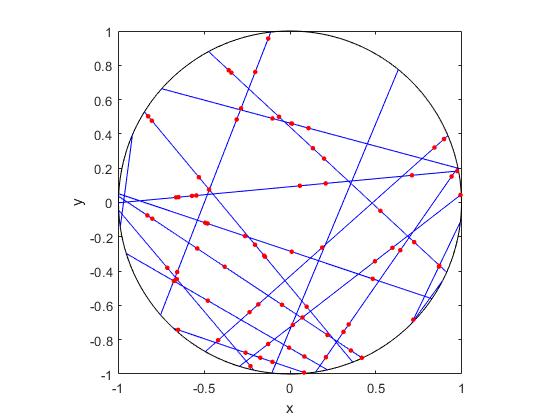##### R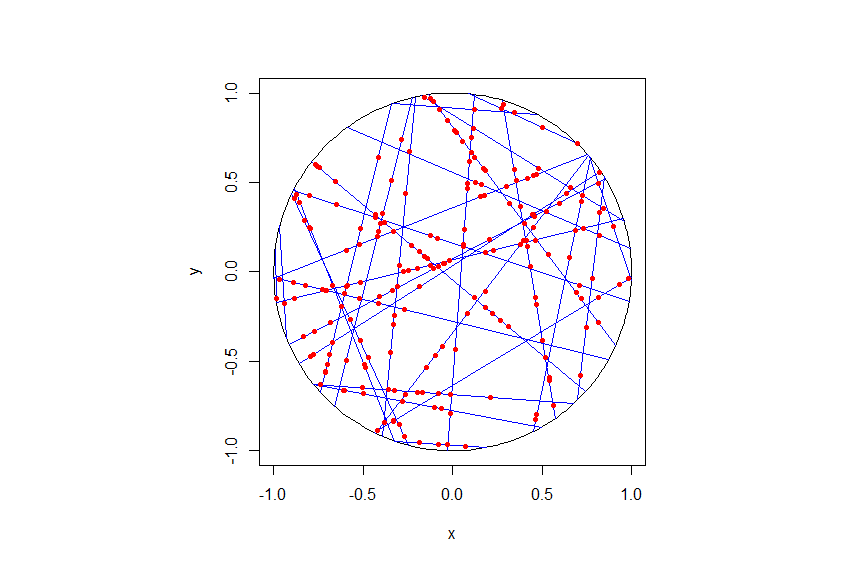##### Python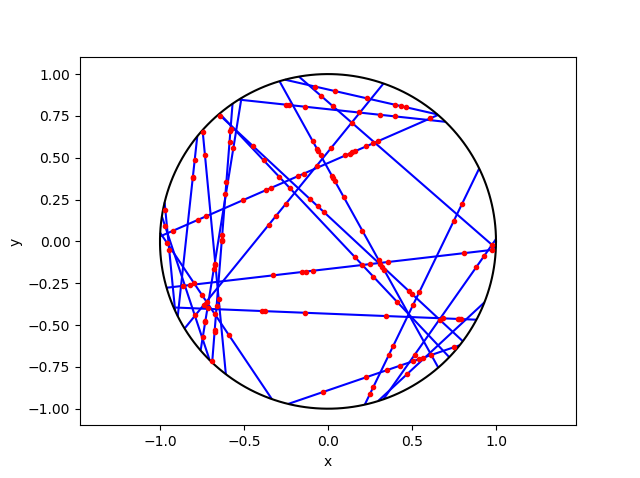## Code

As always, the code from all my posts is online. For this post, I have written the code in MATLAB, R and Python.

For the first step, the reading material is basically the same as that for the Poisson line process, which overlaps with that of the Bertrand paradox. For the one-dimensional Poisson point process, we can use the reading material on the homogeneous Poisson point process on a rectangle.

For general Cox point processes, I recommend starting with the following: Chapter 6 in the monograph Poisson processes by Kingman; Chapter 5 in Statistical Inference and Simulation for Spatial Point Processes by Møller and Waagepetersen; and Section 5.2 in Stochastic Geometry and its Applications by Chiu, Stoyan, Kendall and Mecke. For a much more mathematical treatment, see Chapter 13 in Lectures on the Poisson Process by Last and Penrose.

For this particularly Cox point process, see the two aforementioned preprints, located here and here.

## Simulating a Poisson line process

Instead of points, we can consider other objects randomly scattered on some underlying mathematical space. If we take a Poisson point process, then we can use (infinitely long) straight lines instead of points, giving a Poisson line process. Researchers have studied and used this random object to model physical phenomena. In this post I’ll cover how to simulate a homogeneous Poisson line process in MATLAB, R and Python. The code which can be downloaded from here

## Overview

For simulating a Poisson line process, the key question is how to randomly position the lines.  This is related to a classic problem in probability theory called the Bertrand paradox.  I discussed this illustration in probability in a previous post, where I also included code for simulating it. I highly recommend reading that post first.

The Bertrand paradox involves three methods for positioning random lines. We use Method 2 to achieve a uniform positioning of lines, meaning the number of lines and orientation of lines is statistically uniform. Then it also makes sense to use this method for simulating a homogeneous (or uniform) Poisson line process.

We can interpret a line process as a point process. For a line process on the plane $$\textbf{R}^2$$, it can be described by a point process on $$(0,\infty)\times (0,2\pi)$$, which is an an infinitely long cylinder. In other words, the Poisson line process can be described as a Poisson point process.

For simulating a Poisson line process, it turns out the disk is the most natural setting. (Again, this goes back to the Bertrand paradox.) More specifically, how the (infinitely long) lines intersect a disk of a fixed radius $$r>0$$. The problem of simulating a Poisson line process reduces to randomly placing chords in a disk. For other simulation windows in the plane, we can always bound any non-circular region with a sufficiently large disk.

## Steps

##### Number of lines

Of course, with all things Poisson, the number of lines will be  a Poisson random variable, but what will its parameter be? This homogeneous (or uniform) Poisson line process forms a one-dimensional homogeneous (or uniform) Poisson point process around the edge of the disk with a circumference $$2 \pi r$$. Then the number of lines is simply a Poisson variable with parameter $$\lambda 2 \pi r$$.

##### Locations of points

To position a single line uniformly in a disk, we need to generate two uniform random variables. One random variable gives the angle describing orientation of the line, so it’s a uniform random variable $$\Theta$$ on the interval $$(0,2\pi)$$.

The other random variable gives the distance from the origin to the disk edge, meaning it’s a uniform random variable $$P$$ on the interval $$(0,r)$$, where $$r$$ is the radius of the disk.  The random radius and its perpendicular chord create a right-angle triangle.  The distance from the point $$(\Theta, P)$$ to the disk edge (that is, the circle) along the chord is:

$$Q=\sqrt{r^2-P^2}.$$

The endpoints of the chord (that is, the points on the disk edge) are then:

Point 1: $$X_1=P \cos \Theta+ Q\sin \Theta$$, $$Y_1= P \sin \Theta- Q\cos \Theta$$,

Point 2: $$X_2=P \cos \Theta- Q\sin \Theta$$, $$Y_2= P \sin \Theta+Q \cos \Theta$$.

## Code

I have implemented the simulation procedure in MATLAB, R and Python, which, as usual, are all very similar. I haven’t put my code here, because the software behind my website keeps mangling it.  As always, I have uploaded my code to a repository; for this post, it’s in this directory.

I have written the code in R, but I wouldn’t use it in general. That’s because if you’re using R, then, as I have said before, I strongly recommend using the powerful spatial statistics library spatstat. For a simulating Poisson line process, there’s the function rpoisline.

The chief author of spatstat, Adrian Baddeley, has written various lectures and books on the related topics of point processes, spatial statistics, and geometric probability. In this post, he answered why the angular coordinates have to be chosen uniformly.

### Results

MATLAB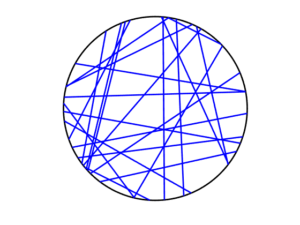R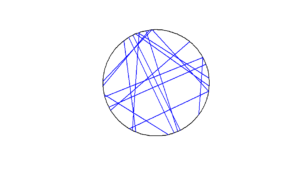Python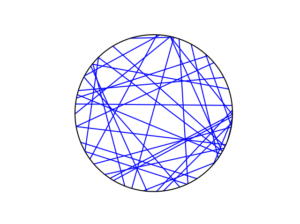To read about the Poisson line process, it’s best to start with the Bertrand problem, which is covered in many works on geometric probability and related fields. A good and recent introduction is given by Calka in (Section 1.3) of the lectures titled Stochastic Geometry: Modern Research Frontiers, which were edited by Coupier and published by Springer.  Also see, for example, problem 1.2 in Geometrical Probability by Kendall and Moran or page 44 in Geometric Probability by Solomon.

For the Poisson line process, I highly recommend Section 7.2 in the monograph Poisson processes by Kingman. Also see Example 8.2 in the standard textbook Stochastic Geometry and its Applications by Chiu, Stoyan, Kendall and Mecke. The Poisson line process book also appears in Exercise 5.2 in the book Stochastic Simulation – Algorithms and Analysis by  Asmussen and Glynn.

For online resources, this set of lectures by Christian Lantuéjoul
covers the Poisson line process. Wilfrid Kendall covers the Poisson line process in this talk in relation to so-called Poisson cities.

Mathematical paradoxes are results or observations in mathematics that are (seemingly) conflicting, unintuitive, incomprehensible, or just plain bizarre. They come in different flavours, such as those that play with notions of infinity, which means they often make little or no sense in a physical world. Other paradoxes, particularly those in probability, serve as a lesson that the problem needs to be posed in a precise manner. The Bertrand paradox is one of these.

Joseph Bertrand posed the original problem in his 1889 book Calcul des probabilités, which is available online (albeit in French). It’s a great illustrative problem involving simple probability and geometry, so it often appears in literature on the (closely related) mathematical fields of geometric probability and integral geometry.

Based on constructing a random chord in a circle, the paradox involves a single mathematical problem with three reasonable but different solutions. It’s less a paradox and more a cautionary tale. It’s really asking the question: What do you mean by random?

Consequently, over the years the Bertrand paradox has inspired debate, with papers arguing what the true solution is. I recently discovered it has even inspired some passionate remarks on the internet; read the comments at www.bertrands-paradox.com.

But I am less interested in the different interpretations or philosophies of the problem. Rather, I want to simulate the three solutions. This is not very difficult, provided some trigonometry and knowledge from a previous post, where I describe how to simulate a (homogeneous) Poisson point process on the disk.

I won’t try to give a thorough analysis of the solutions, as there are much better websites doing that. For example, this MIT website gives a colourful explanation with pizza and fire-breathing monsters. The Wikipedia article also gives a detailed though less creative explanation for the three solutions.

My final code in MATLAB, R and Python code is located here.

### The Problem

Bertrand considered a circle with an equilateral triangle inscribed it. If a chord in the circle is randomly chosen, what is the probability that the chord is longer than a side of the equilateral triangle?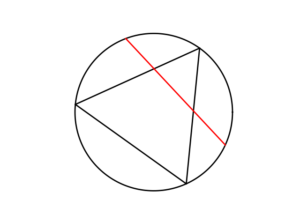### The Solution(s)

Bertrand argued that there are three natural but different methods to randomly choose a chord, giving three distinct answers. (Of course, there are other methods, but these are arguably not the natural ones that first come to mind.)

##### Method 1: Random endpoints

On the circumference of the circle two points are randomly (that is, uniformly and independently) chosen, which are then used as the two endpoints of the chord.

The probability of this random chord being longer than a side of the triangle is one third.

A radius of the circle is randomly chosen (so the angle is chosen uniformly), then a point is randomly (also uniformly) chosen along the radius, and then a chord is constructed at this point so it is perpendicular to the radius.

The probability of this random chord being longer than a side of the triangle is one half.

##### Method 3: Random midpoint

A point is randomly (so uniformly) chosen in the circle, which is used as the midpoint of the chord, and the chord is randomly (also uniformly) rotated.

The probability of this random chord being longer than a side of the triangle is one quarter.

### Simulation

All three answers involve randomly and independently sampling two random variables, and then doing some simple trigonometry. The setting naturally inspires the use of polar coordinates. I assume the circle has a radius $$r$$ and a centre at the point origin $$o$$. I’ll number the end points one and two.

In all three solutions we need to generate uniform random variables on the interval $$(0, 2\pi)$$ to simulate random angles. I have already done this a couple of times in previous posts such as this one.

##### Method 1: Random endpoints

This is probably the most straightforward solution to simulate. We just need to simulate two uniform random variables $$\Theta_1$$ and $$\Theta_2$$ on the interval $$(0, 2\pi)$$ to describe the angles of the two points.

The end points of the chord (in Cartesian coordinates) are then simply:

Point 1: $$X_1=r \cos \Theta_1$$, $$Y_1=r \sin \Theta_1$$,

Point 2: $$X_2=r \cos \Theta_2$$, $$Y_2=r \sin \Theta_2$$.

This method also involves generating two uniform random variables. One random variable $$\Theta$$ is for the angle, while the other $$P$$ is the random radius, which means generating the random variable $$P$$ on the interval $$(0, r)$$.

I won’t go into the trigonometry, but the random radius and its perpendicular chord create a right-angle triangle. The distance from the point $$(\Theta, P)$$ to the circle along the chord is:

$$Q=\sqrt{r^2-P^2}.$$

The endpoints of the chord are then:

Point 1: $$X_1=P \cos \Theta+ Q\sin \Theta$$, $$Y_1= P \sin \Theta- Q\cos \Theta$$,

Point 2: $$X_2=P \cos \Theta- Q\sin \Theta$$, $$Y_2= P \sin \Theta+Q \cos \Theta$$.

Take note of the signs in these expressions.

##### Method 3: Random midpoint

This method requires placing a point uniformly on a disk, which is also done when simulating a homogeneous Poisson point process on a disk, and requires two random variables $$\Theta’$$ and $$P’$$. Again, the angular random variable $$\Theta’$$ is uniform.

The other random variable $$P’$$ is not uniform. For $$P’$$, we generate a random uniform variable on the unit interval $$(0,1)$$, and then we take the square root of it. We then multiply it by the radius, generating a random variable between $$0$$ and $$r$$. (We must take the square root because the area element of a sector is proportional to the radius squared, and not the radius.) The distribution of this random variable is an example of the triangular distribution.

The same trigonometry from Method 2 applies here, which gives the endpoints of the chord as:

Point 1: $$X_1=P’ \cos \Theta’+ Q’\sin \Theta’$$, $$Y_1= P’ \sin \Theta’- Q’\cos \Theta’$$,

Point 2: $$X_2=P’\cos \Theta’- Q’\sin \Theta’$$, $$Y_2= P’\sin \Theta’+Q’ \cos \Theta’$$,

where $$Q’:=\sqrt{r^2-{P’}^2}$$. Again, take note of the signs in these expressions.

### Results

To illustrate how the three solutions are different, I’ve plotted a hundred random line segments and their midpoints side by side. Similar plots are in the Wikipedia article.

#####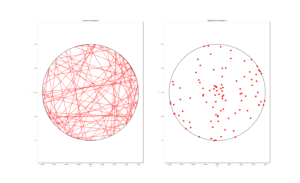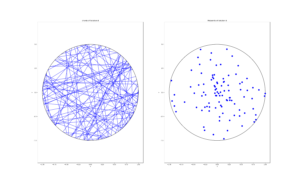##### Method 3: Random midpoint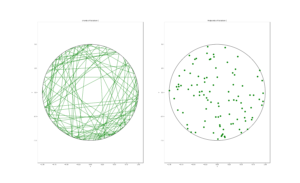### Conclusion

For the chord midpoints, we know and can see that Method 3 gives uniform points, while Method 2 has a concentration of midpoints around the circle centre. Method 1 gives results that seem to somewhere between Method 2 and 3 in terms of clustering around the circle centre.

For the chords, we see that Method 3 results in fewer chords passing through the circle centre. Methods 1 and 2 seem to give a similar number of lines passing through this central region.

It’s perhaps hard to see, but it can be shown that Method 2 gives the most uniform results. By this, I mean that the number of lines and their orientations statistically do not vary in different regions of the circle.

We can now position random lines in uniform manner. All we need now is a Poisson number of lines to generate something known as a Poisson line process, which will be the focus of the next post.

I’ve already mentioned that there are some good websites on the topic of the Bertrand paradox. For example:

web.mit.edu/tee/www/bertrand

www.cut-the-knot.org/bertrand.shtml

mathworld.wolfram.com/BertrandsProblem.html

Various authors have mentioned or discussed the Bertrand paradox in books on the related subjects of geometric probability, integral geometry and stochastic geometry. A good and recent introduction is given by Calka in Section 1.3 of the published lectures Stochastic Geometry: Modern Research Frontiers.

Other classic books that cover the topic including, for example, see Problem 1.2 in Geometrical Probability by Kendall and Moran. (Despite Maurice G. Kendall writing a book on geometric probability, he was not related to stochastic geometry pioneer David G Kendall.) It’s also discussed on page 44 in Geometric Probability by Solomon. For a book that involves more advance knowledge of geometry and (abstract) algebra, see Chapter 3 in Integral Geometry and Geometric Probability by Santaló.

The Bertrand paradox is also in The Pleasures of Probability by Isaac. It’s covered in a non-mathematical way in the book Paradoxes from A to Z by Clark. Edwin Jaynes studied the problem and proposed a solution in a somewhat famous 1973 paper, titled The Well-Posed Problem.

The original problem can be read in French in Bertrand’s work, which is available online here or here (starting at the bottom of page 4).

### Code

The MATLAB, R and Python code can be found here. In the code, I have labelled the methods A, B and C instead 1, 2 and 3.

## Testing the Julia language with point process simulations

I started writing these posts (or blog entries) about a year ago. In my first post I remarked how I wanted to learn to write stochastic simulations in a new language. Well, I found one. It’s called Julia. Here’s my code. And here are my thoughts.

### Overview

For scientific programming, the Julia language has arisen as a new contender. Originally started in 2012, its founders and developers have (very) high aspirations, wanting the language to be powerful and accessible, while still having run speeds comparable to C. There’s been excitement about it, and even a Nobel Laureate in economics, Thomas Sargent, has endorsed it. He co-founded the QuantEcon project, whose website has this handy guide or cheat sheet for commands between MATLAB, Python and Julia.

That guide suggests that Julia’s main syntax inspiration comes from MATLAB. But perhaps its closest (and greatest) competitor in scientific programming languages is Python, which has become a standard language used in scientific programming, particularly in machine learning. Another competitor is the statistics language R, which is popular for data science. But R is not renown for its speed.

As an aside, machine learning is closely related to what many call data science. I consider the two disciplines as largely overlapping with statistics, where their respective emphases are on theory and practice. In these fields, often the languages Python and R are used. There are various websites discussing which language is better, such as this one, which in turn is based on this one. In general, it appears that computer scientists and statisticians respectively prefer using Python and R.

Returning to the Julia language, given its young age, the language is still very much evolving, but I managed to find suitable Julia functions for stochastic simulations. I thought I would try it out by simulating some point processes, which I have done several times before. I successfully ran all my code with Julia Version 1.0.3.

In short, I managed to replicate in (or even translate to) Julia the code that I presented in the following posts:

Simulating a homogeneous Poisson point process on a rectangle

Simulating a Poisson point process on a disk

Simulating a Poisson point process on a triangle

Simulating an inhomogeneous Poisson point process

Simulating a Matérn cluster point process

Simulating a Thomas cluster point process

The Julia code, like all the code I present here, can be found on my Github repository, which for this post is located here.

### Basics

##### Language type and syntax

The Wikipedia article on Julia says:

Julia is a high-level general-purpose dynamic programming language designed for high-performance numerical analysis and computational science.

Scientific programming languages like the popular three MATLAB, R and Python, are interpreted languages. But the people behind Julia say:

it is a flexible dynamic language, appropriate for scientific and numerical computing, with performance comparable to traditional statically-typed languages.

Because Julia’s compiler is different from the interpreters used for languages like Python or R, you may find that Julia’s performance is unintuitive at first.

I already remarked that Julia’s syntax is clearly inspired by MATLAB, as one can see in this guide for MATLAB, Python and Julia. But there are key differences. For example, to access an array entry in Julia, you use square brackets (like in most programming languages), whereas parentheses are used in MATLAB.

##### Packages

Julia requires you to install certain packages or libraries, like most languages. For random simulations and plots, you have to install the Julia packages Distributions and Plots, which is done by running the code.

Pkg.add("Distributions");
Pkg.add("Plots");

After doing that, it’s best to restart Julia. These packages are loaded with the using command:

Using Distributions;
Using Plots;

Also, the first time it takes a while to run any code using those newly installed packages.

I should stress that there are different plotting libraries, but Plots, which contains many plotting libraries, is the only one I could get working. Another is PlotPy, which uses the Python library. As a beginner, it seems to me that the Julia community has not focused too much on developing new plotting functions, and has instead leveraged pre-existing libraries.

For standard scientific and statistical programming, you will usually also need the packages LinearAlgebra and Statistics.

##### Data types

Unlike MATLAB or R, Julia is a language that has different data types for numbers, such as integers and floating-point numbers (or floats). This puts Julia in agreement with the clear majority of languages, making it nothing new for most programmers. This is not a criticism of the language, but this can be troublesome if you’ve grown lazy after years of using MATLAB and R.

##### Simulating random variables

In MATLAB, R and Python, we just need to call a function for simulating uniform, Poisson, and other random variables. There’s usually a function for each type of random variable (or probability distribution).

Julia does simulation of random objects in a more, let’s say, object-oriented way (but I’m told, it’s not an object-oriented language). The probability distributions of random variables are objects, which are created and then sent to a general function for random generation. For example, here’s the code for simulating a Poisson variable with mean $$\mu=10$$.

mu=10;
distPoisson=Poisson(mu);
numbPoisson=rand(distPoisson);

Similarly, here’s how to simulate a normal variable with mean $$\mu=10$$ and standard deviation $$\sigma=1$$.

sigma=1;
distNormal=Normal(mu,sigma);
numbNormal=rand(distNormal);

Of course the last two lines can be collapsed into one.

mu=10;
sigma=1;
numbNormal=rand(Normal(mu,sigma));

But if you just want to create standard uniform variables on the interval (0,1), then the code is like that in MATLAB. For example, this code creates a $$4\times3$$ matrix (or array) $$X$$ whose entries are simulation outcomes of independent uniform random variables:

X=rand(4,3);

The resulting matrix $$X$$ is a Float 64 array.

##### Arrays

The indexing of arrays in Julia starts at one, like MATLAB and R. When you apply a function to an array, you generally need to use the dot notation. For example, if I try to run the code:

Y=sqrt(rand(10,1)); #This line will result in an error.

then on my machine (with Julia Version 1.0.3) I get the error:

ERROR: DimensionMismatch(“matrix is not square: dimensions are (10, 1)”)

But this code works:

Y=sqrt.(rand(10,1));

Also, adding scalars to arrays can catch you in Julia, as you also often need to use the dot notation. This code:

Y=sqrt.(rand(10,1));
Z=Y+1; #This line will result in an error.

gives the error:

ERROR: MethodError: no method matching +(::Array{Float64,2}, ::Int64)

This is fixed by adding a dot:

Y=sqrt.(rand(10,1));
Z=Y.+1; #This line will work.

Note the dot has to be on the left hand side. I ended up just using dot notation every time to be safe.

Other traps exist. For example, with indexing, you need to convert floats to integers if you want to use them as indices.

##### Repeating array elements

There used to be a Julia function called repmat, like the one in MATLAB , but it was merged with a function called repeat. I used such repeating operations to avoid explicit for-loops, which is generally advised in languages like MATLAB and R. For example, I used the repelem function in MATLAB to simulate Matérn and Thomas cluster point processes. To do this in Julia, I had to use this nested construction:

y=vcat(fill.(x, n)...);

This line means that the first value in $$x$$ is repeated $$n$$ times, where $$n$$ is the first entry of $$n$$ (as indexing in Julia starts at one), then the second value of $$x$$ is repeated $$n$$ times, and so on. For example, the vectors $$x=[7,4,9]$$ and $$n=[2,1,3]$$, the answer is $$y=[7,7,4,9,9,9]$$.

To do this in Julia, the construction is not so bad, if you know how, but it’s not entirely obvious. In MATLAB I use this:

y=repelem(x,n);

Similarly in Python:

y=np.repeat(x,n);
##### Different versions of Julia

I found that certain code would work (or not work) and then later the same code would not work (or would work) on machines with different versions of Julia, demonstrating how the language is still being developed. More specifically, I ran code on Julia Version 1.0.3 (Date 2018-12-18) and Julia Version 0.6.4 (Date: 2018-07-09). (Note how there’s only a few months difference in the dates of the two versions.)

Consider the code with the errors (due to the lack of dot operator) in the previous section. The errors occurred on one machine with Julia Version 1.0.3, but the errors didn’t occur on another machine with the older Julia Version 0.6.4. For a specific example, the code:

Y=sqrt.(rand(10,1));
Z=Y+1; #This line will not result in an error on Version 0.6.4.

gives no error with Julia Version 0.6.4, while I have already discussed how it gives an error with Julia Version 1.0.3.

For another example, I copied from this MATLAB-Python-Julia guide the following command:

A = Diagonal([1,2,3]); #This line will (sometimes?) result in an error.

It runs on machine with Julia Version 0.6.4 with no problems. But running it on the machine with Julia Version 1.0.3 gives the error:

ERROR: UndefVarError: Diagonal not defined

That’s because I have not used the LinearAlgebra package. Fixing this, the following code:

using LinearAlgebra; #Package needed for Diagonal command.
A = Diagonal([1,2,3]); #This line should now work.

gives no error with Julia Version 1.0.3.

If you have the time and energy, you can search the internet and find online forums where the Julia developers have discussed why they have changed something, rendering certain code unworkable with the latest versions of Julia.

##### Optimization

It seems that performing optimization on functions is done with the Optim package.

Pkg.add("Optim");

But some functions need the Linesearches package, so it’s best to install that as well.

Pkg.add("Linesearches");

Despite those two optimization packages, I ended up using yet another package called BlackBoxOptim.

Pkg.add("BlackBoxOptim");

In this package, I used a function called bboptimize. This is the first optimziation function that I managed to get working. I do not know how it compares to the functions in the Optim and Linesearches packages.

In a previous post, I used optimization functions to simulate a inhomogeneous or nonhomogeneous Poisson point process on a rectangle. I’ve also written Julia code for this simulation, which is found below. I used bboptimize, but I had some problems when I initially set the search regions to integers, which the package did not like, as the values need to be floats. That’s why I multiple the rectangle dimensions by $$1.0$$ in the following code:

boundSearch=[(1.0xMin,1.0xMax), (1.0yMin, 1.0yMax)]; #bounds for search box
#WARNING: Values of boundSearch cannot be integers!
resultsOpt=bboptimize(fun_Neg;SearchRange = boundSearch);
lambdaNegMin=best_fitness(resultsOpt); #retrieve minimum value found by bboptimize

## Conclusion

In this brief experiment, I found the language Julia good for doing stochastic simulations, but too tricky for doing simple things like plotting. Also, depending on the version of Julia, sometimes my code would work and sometimes it wouldn’t. No doubt things will get better with time.

As I said, Julia is still very much an ongoing project. Here’s a couple of links that helped me learn the basics.

https://en.wikibooks.org/wiki/Introducing_Julia/Arrays_and_tuples

https://voxeu.org/content/which-numerical-computing-language-best-julia-matlab-python-or-r

Julia, Matlab, and C

https://modelingguru.nasa.gov/docs/DOC-2676

## Code

I’ve only posted here code for some of simulations, but the rest of the code is available on my GitHub repository located here. You can see how the code is comparable to that of MATLAB.

##### Poisson point process on a rectangle

using Distributions #for random simulations
using Plots #for plotting

#Simulation window parameters
xMin=0;xMax=1;
yMin=0;yMax=1;
xDelta=xMax-xMin;yDelta=yMax-yMin; #rectangle dimensions
areaTotal=xDelta*yDelta;

#Point process parameters
lambda=100; #intensity (ie mean density) of the Poisson process

#Simulate Poisson point process
numbPoints=rand(Poisson(areaTotal*lambda)); #Poisson number of points
xx=xDelta*rand(numbPoints,1).+xMin;#x coordinates of Poisson points
yy=yDelta*(rand(numbPoints,1)).+yMin;#y coordinates of Poisson points

#Plotting
plot1=scatter(xx,yy,xlabel ="x",ylabel ="y", leg=false);
display(plot1);
##### Inhomogeneous Poisson point process on a rectangle

using Distributions #for random simulations
using Plots #for plotting
using BlackBoxOptim #for blackbox optimizing

#Simulation window parameters
xMin=-1;xMax=1;
yMin=-1;yMax=1;
xDelta=xMax-xMin;yDelta=yMax-yMin; #rectangle dimensions
areaTotal=xDelta*yDelta;

s=0.5; #scale parameter

#Point process parameters
function fun_lambda(x,y)
100*exp.(-(x.^2+y.^2)/s^2); #intensity function
end

###START -- find maximum lambda -- START ###
#For an intensity function lambda, given by function fun_lambda,
#finds the maximum of lambda in a rectangular region given by
#[xMin,xMax,yMin,yMax].
#NOTE: Need xMin, xMax, yMin, yMax to be floats eg xMax=1. See boundSearch

function fun_Neg(x)
-fun_lambda(x,x); #negative of lambda
end
xy0=[(xMin+xMax)/2.0,(yMin+yMax)/2.0];#initial value(ie centre)

#Find largest lambda value
boundSearch=[(1.0xMin,1.0xMax), (1.0yMin, 1.0yMax)];
#WARNING: Values of boundSearch cannot be integers!
resultsOpt=bboptimize(fun_Neg;SearchRange = boundSearch);
lambdaNegMin=best_fitness(resultsOpt); #retrieve minimum value found by bboptimize
lambdaMax=-lambdaNegMin;
###END -- find maximum lambda -- END ###

#define thinning probability function
function fun_p(x,y)
fun_lambda(x,y)/lambdaMax;
end

#Simulate a Poisson point process
numbPoints=rand(Poisson(areaTotal*lambdaMax)); #Poisson number of points
xx=xDelta*rand(numbPoints,1).+xMin;#x coordinates of Poisson points
yy=yDelta*(rand(numbPoints,1)).+yMin;#y coordinates of Poisson points

#calculate spatially-dependent thinning probabilities
p=fun_p(xx,yy);
#Generate Bernoulli variables (ie coin flips) for thinning
booleRetained=rand(numbPoints,1).<p; #points to be retained
xxRetained=xx[booleRetained]; yyRetained=yy[booleRetained];

#Plotting
plot1=scatter(xxRetained,yyRetained,xlabel ="x",ylabel ="y", leg=false);
display(plot1);
##### Thomas point process on a rectangle

using Distributions #for random simulations
using Plots #for plotting

#Simulation window parameters
xMin=-.5;
xMax=.5;
yMin=-.5;
yMax=.5;

#Parameters for the parent and daughter point processes
lambdaParent=10;#density of parent Poisson point process
lambdaDaughter=10;#mean number of points in each cluster
sigma=0.05; #sigma for normal variables (ie random locations) of daughters

#Extended simulation windows parameters
rExt=7*sigma; #extension parameter
#for rExt, use factor of deviation sigma eg 6 or 7
xMinExt=xMin-rExt;
xMaxExt=xMax+rExt;
yMinExt=yMin-rExt;
yMaxExt=yMax+rExt;
#rectangle dimensions
xDeltaExt=xMaxExt-xMinExt;
yDeltaExt=yMaxExt-yMinExt;
areaTotalExt=xDeltaExt*yDeltaExt; #area of extended rectangle

#Simulate Poisson point process
numbPointsParent=rand(Poisson(areaTotalExt*lambdaParent)); #Poisson number of points

#x and y coordinates of Poisson points for the parent
xxParent=xMinExt.+xDeltaExt*rand(numbPointsParent,1);
yyParent=yMinExt.+yDeltaExt*rand(numbPointsParent,1);

#Simulate Poisson point process for the daughters (ie final poiint process)
numbPoints=sum(numbPointsDaughter); #total number of points

#Generate the (relative) locations in Cartesian coordinates by
#simulating independent normal variables
xx0=rand(Normal(0,sigma),numbPoints);
yy0=rand(Normal(0,sigma),numbPoints);

#replicate parent points (ie centres of disks/clusters)
xx=vcat(fill.(xxParent, numbPointsDaughter)...);
yy=vcat(fill.(yyParent, numbPointsDaughter)...);

#Shift centre of disk to (xx0,yy0)
xx=xx.+xx0;
yy=yy.+yy0;

#thin points if outside the simulation window
booleInside=((xx.>=xMin).&(xx.<=xMax).&(yy.>=yMin).&(yy.<=yMax));
#retain points inside simulation window
xx=xx[booleInside];
yy=yy[booleInside];

#Plotting
plot1=scatter(xx,yy,xlabel ="x",ylabel ="y", leg=false);
display(plot1);

## Checking Poisson point process simulations

In previous posts I described how to simulate homogeneous Poisson point processes on a rectangledisk and triangle. Then I covered how to randomly thin a point process in a spatially dependent manner. Building off these posts, I  wrote in my last post how to simulate an inhomogeneous or nonhomogeneous Poisson point process. Now I’ll describe how to verify that the simulation code correctly simulates the desired Poisson point process.

I should add that although this post is focused on the Poisson point process, parts of the material hold for all point processes. Also, not surprisingly, some of the material and the code overlaps with that presented in the post on the inhomogeneous point process.

## Basics

Any Poisson point process is defined with a measure called the intensity measure or mean measure, which I’ll denote by $$\Lambda$$.  For practical purposes, I assume that the intensity measure $$\Lambda$$ has a derivative $$\lambda(x,y)$$, where I have used Cartesian coordinates.  The function $$\lambda(x,y)$$ is often called the intensity function or just intensity, which I  assume is bounded, so $$\lambda(x,y)<\infty$$ for all points in a simulation window $$W$$. Finally, I assume that the simulation window $$W$$ is a rectangle.

Several times before I have mentioned that simulating a Poisson point process requires simulating two random components: the number of points and the locations of points.  Working backwards, to check a Poisson simulation, we must run the Poisson simulation a large number of times (say $$10^3$$ or $$10^4$$), and collect the statistics on these two properties. We’ll start by examining the easiest of the two.

## Number of points

For any Poisson point process, the number of points is a Poisson random variable with a parameter (that is, a mean) $$\Lambda(W)$$, which under our previous assumptions is given by the surface integral

$$\Lambda(W)=\int_W \lambda(x,y)dxdy.$$

Presumably we can evaluate such an integral analytically or numerically in order to simulate the Poisson point process. To check that we correctly simulate the random the number of points, we just need to simulate the point process a large number  of times, and compare the statistics to those given by the analytic expressions.

##### Moments

The definition of the intensity measure of a point process is a measure that gives the average or expected number of points in some region. As the number of simulations increases, the (sample) average number of points will converge to the intensity measure $$\Lambda(W)$$.  I should stress that this is a test for the intensity measure, a type of first moment, which will work for the intensity measure of any point process.

For Poisson point processes, there is another moment test that can be done.  It can be shown mathematically that the variance of the number of points will also converge to the intensity measure $$\Lambda(W)$$, giving a second empirical test based on second moments. There is no pointp process theory here, as this moment result is simply due to the number of points being distributed according to a Poisson distribution. The second moment is very good for checking Poissonness, forming the basis for statistical tests. If this and the first moment test hold, then there’s a strong chance the number of points is a Poisson variable.

##### Empirical distribution

Beyond the first two moments, an even more thorough test is to estimate an empirical distribution for the number of points. That is, we perform a histogram on the number of points, and then we normalize it, so the total mass sums to one, producing an empirical probability distribution for the number of points.

The results should closely match the probability mass function of a Poisson distribution with parameter $$\Lambda(W)$$, namely

$$P(N=n)= \frac{[\Lambda(W)]^n}{n!} e^{-\Lambda(W)} ,$$

where $$N$$ is the random number of points in the window $$W$$, which has the area $$|W|$$. If the empirical distribution is close to results given by the above expression, then we can confidently say that the number of points is a Poisson random variable with the right parameter or mean.

## Locations of points

To check the positioning of points, we can empirically estimate the intensity function $$\lambda(x,y)$$. A simple way to do this is to perform a two-dimensional histogram on the point locations. This is very similar to the one-dimensional histogram, which I suggested to do for testing the number of points. It just involves counting the number of points that fall into two-dimensional non-overlapping subsets called bins.

To estimate the intensity function, each bin count needs to be rescaled by the area of the bin, giving a density value for each bin. This empirical estimate of the intensity function should resemble the true intensity function $$\lambda(x,y)$$. For a visual comparison, we can use a surface plot to illustrate the two sets of results.

This procedure will work for estimating the intensity function of any point process, not just a Poisson one.

In spatial statistics, there are more advanced statistical tests for testing how Poisson a point pattern is.  But these tests are arguably too complicated for checking a simple point process that is rather easy to simulate. Furthermore, researchers usually apply these tests to a small number of point patterns. In this setting, it is not possible to accurately obtain empirical distributions without further assumptions. But with simulations, we can generate many simulations and obtain good empirical distributions. In short, I would never use such tests for just checking that I have properly coded a Poisson simulation.

## Results

I produced the results with ten thousand simulations, which gave good results and took only a few seconds to complete on a standard desktop computer. Clearly increasing the number of simulations increases the accuracy of the statistics, but it also increases the computation time.

For the results, I used the intensity function

$$\lambda(x,y)=\lambda_1(x,y)+\lambda_2(x,y),$$

where

$$\lambda_1(x,y)=80e^{-((x+0.5)^2+(y+0.5)^2)/s^2},$$

$$\lambda_2(x,y)=100e^{-((x-0.5)^2+(y-0.5)^2)/s^2},$$

and $$s>0$$ is a scale parameter. We can see that this function has two maxima or peaks at $$(-0.5,-0.5)$$ and $$(0.5,0.5)$$.

##### MATLAB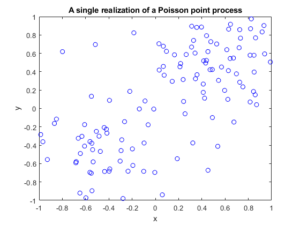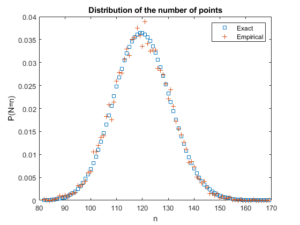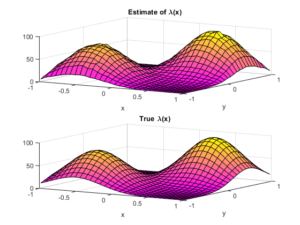##### Python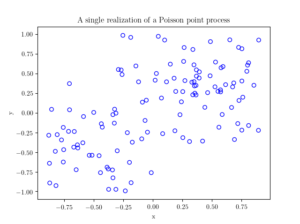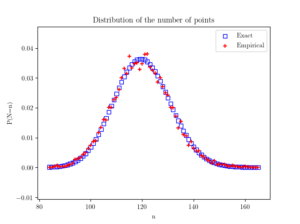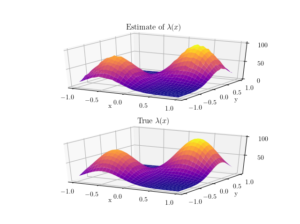I have not covered much new theoretical stuff in this post, so looking at the references in previous posts, such as this one, should help.

For two-dimensional histograms, I recommend going to the respective MATLAB and Python function websites.  Here’s an example of a two-dimensional histogram implemented in Python.

Probably the most difficult part for me was performing the plotting in Python.  I recommend these links:

https://plot.ly/python/3d-surface-plots/

https://chrisalbon.com/python/basics/set_the_color_of_a_matplotlib/

https://matplotlib.org/examples/color/colormaps_reference.html

## Code

All code from my posts, as always, can be found on the my GitHub repository. The code for this post is located here.

The estimating the statistical moments is standard. Performing the histograms is also routine, but when normalizing, you have to choose the option that returns the empirical estimate of the probability density function (pdf).

Fortunately, scientific programming languages usually have functions for performing two-dimensional histograms. What is  a bit tricky is how to normalize or rescale the bin counts. The histogram functions can,  for example, divide by the number of simulations, the area of each bin, or both. In the end, I chose the pdf option  in both MATLAB and Python to give an empirical estimate of the probability density function, and then multiplied it by the average number of points, which was calculated in the previous check. (Although,  I could have done this in a single step in MATLAB, but not in Python, so I chose to do it in a couple of steps in both languages so the code matches more closely.)

##### MATLAB

I used the surf function to plot the intensity function and its estimate; see below for details on the histograms.

close all;

%Simulation window parameters
xMin=-1;xMax=1;
yMin=-1;yMax=1;
xDelta=xMax-xMin;yDelta=yMax-yMin; %rectangle dimensions
areaTotal=xDelta*yDelta; %area of rectangle

numbSim=10^4; %number of simulations

s=0.5; %scale parameter

%Point process parameters
fun_lambda=@(x,y)(100*exp(-((x).^2+(y).^2)/s^2));%intensity function

%%%START -- find maximum lambda -- START %%%
%For an intensity function lambda, given by function fun_lambda,
%finds the maximum of lambda in a rectangular region given by
%[xMin,xMax,yMin,yMax].
funNeg=@(x)(-fun_lambda(x(1),x(2))); %negative of lambda
%initial value(ie centre)
xy0=[(xMin+xMax)/2,(yMin+yMax)/2];%initial value(ie centre)
%Set up optimization step
options=optimoptions('fmincon','Display','off');
%Find largest lambda value
[~,lambdaNegMin]=fmincon(funNeg,xy0,[],[],[],[],...
[xMin,yMin],[xMax,yMax],'',options);
lambdaMax=-lambdaNegMin;
%%%END -- find maximum lambda -- END%%%

%define thinning probability function
fun_p=@(x,y)(fun_lambda(x,y)/lambdaMax);

%for collecting statistics -- set numbSim=1 for one simulation
numbPointsRetained=zeros(numbSim,1); %vector to record number of points
for ii=1:numbSim
%Simulate Poisson point process
numbPoints=poissrnd(areaTotal*lambdaMax);%Poisson number of points
xx=xDelta*(rand(numbPoints,1))+xMin;%x coordinates of Poisson points
yy=xDelta*(rand(numbPoints,1))+yMin;%y coordinates of Poisson points

%calculate spatially-dependent thinning probabilities
p=fun_p(xx,yy);

%Generate Bernoulli variables (ie coin flips) for thinning
booleRetained=rand(numbPoints,1)<p; %points to be retained %x/y locations of retained points xxRetained=xx(booleRetained); yyRetained=yy(booleRetained); %collect number of points simulated numbPointsRetained(ii)=length(xxRetained); end %Plotting plot(xxRetained,yyRetained,'bo'); %plot retained points xlabel('x');ylabel('y'); %run empirical test on number of points generated if numbSim&gt;=10
%total mean measure (average number of points)
LambdaNumerical=integral2(fun_lambda,xMin,xMax,yMin,yMax)
%Test: as numbSim increases, numbPointsMean converges to LambdaNumerical
numbPointsMean=mean(numbPointsRetained)
%Test: as numbSim increases, numbPointsVar converges to LambdaNumerical
numbPointsVar=var(numbPointsRetained)

end


For the histogram section, I used the histcounts and histcounts2 functions respectively to estimate the distribution of the number of points and the intensity function. I used the pdf option.

Number of points

histcounts(numbPointsRetained,binEdges,'Normalization','pdf');


Locations of points

histcounts2(xxVectorAll,yyVectorAll,numbBins,'Normalization','pdf');

##### Python

I used the Matplotlib library to plot the intensity function and its estimate; see below for details on the histograms.

import numpy as np; #NumPy package for arrays, random number generation, etc
import matplotlib.pyplot as plt #for plotting
from matplotlib import cm #for heatmap plotting
from mpl_toolkits import mplot3d #for 3-D plots
from scipy.optimize import minimize #for optimizing
from scipy import integrate #for integrating
from scipy.stats import poisson #for the Poisson probability mass function

plt.close("all"); #close all plots

#Simulation window parameters
xMin=-1;xMax=1;
yMin=-1;yMax=1;
xDelta=xMax-xMin;yDelta=yMax-yMin; #rectangle dimensions
areaTotal=xDelta*yDelta;

numbSim=10**4; #number of simulations
numbBins=30; #number of bins for histogram

#Point process parameters
s=0.5; #scale parameter

def fun_lambda(x,y):
#intensity function
lambdaValue=80*np.exp(-((x+0.5)**2+(y+0.5)**2)/s**2)+100*np.exp(-((x-0.5)**2+(y-0.5)**2)/s**2);
return lambdaValue;

###START -- find maximum lambda -- START ###
#For an intensity function lambda, given by function fun_lambda,
#finds the maximum of lambda in a rectangular region given by
#[xMin,xMax,yMin,yMax].
def fun_Neg(x):
return -fun_lambda(x,x); #negative of lambda

xy0=[(xMin+xMax)/2,(yMin+yMax)/2];#initial value(ie centre)
#Find largest lambda value
resultsOpt=minimize(fun_Neg,xy0,bounds=((xMin, xMax), (yMin, yMax)));
lambdaNegMin=resultsOpt.fun; #retrieve minimum value found by minimize
lambdaMax=-lambdaNegMin;
###END -- find maximum lambda -- END ###

#define thinning probability function
def fun_p(x,y):
return fun_lambda(x,y)/lambdaMax;

#for collecting statistics -- set numbSim=1 for one simulation
numbPointsRetained=np.zeros(numbSim); #vector to record number of points
xxAll=[]; yyAll=[];

### START -- Simulation section -- START ###
for ii in range(numbSim):
#Simulate a Poisson point process
numbPoints = np.random.poisson(lambdaMax*areaTotal);#Poisson number of points
xx = xDelta*np.random.uniform(0,1,numbPoints)+xMin;#x coordinates of Poisson points
yy = yDelta*np.random.uniform(0,1,numbPoints)+yMin;#y coordinates of Poisson points

#calculate spatially-dependent thinning probabilities
p=fun_p(xx,yy);

#Generate Bernoulli variables (ie coin flips) for thinning
booleRetained=np.random.uniform(0,1,numbPoints)<p; #points to be thinned

#x/y locations of retained points
xxRetained=xx[booleRetained]; yyRetained=yy[booleRetained];
numbPointsRetained[ii]=xxRetained.size;
xxAll.extend(xxRetained); yyAll.extend(yyRetained);
### END -- Simulation section -- END ###

#Plotting a simulation
fig1 = plt.figure();
plt.scatter(xxRetained,yyRetained, edgecolor='b', facecolor='none');
plt.xlabel("x"); plt.ylabel("y");
plt.title('A single realization of a Poisson point process');
plt.show();

#run empirical test on number of points generated
###START -- Checking number of points -- START###
#total mean measure (average number of points)
#Test: as numbSim increases, numbPointsMean converges to LambdaNumerical
numbPointsMean=np.mean(numbPointsRetained);
#Test: as numbSim increases, numbPointsVar converges to LambdaNumerical
numbPointsVar=np.var(numbPointsRetained);
binEdges=np.arange(numbPointsRetained.min(),(numbPointsRetained.max()+1))-0.5;
pdfEmp, binEdges=np.histogram(numbPointsRetained, bins=binEdges,density=True);

nValues=np.arange(numbPointsRetained.min(),numbPointsRetained.max());
#analytic solution of probability density
pdfExact=(poisson.pmf(nValues,LambdaNumerical));

#Plotting
fig2 = plt.figure();
plt.scatter(nValues,pdfExact, color='b', marker='s',facecolor='none',label='Exact');
plt.scatter(nValues,pdfEmp, color='r', marker='+',label='Empirical');
plt.xlabel("n"); plt.ylabel("P(N=n)");
plt.title('Distribution of the number of points');
plt.legend();
plt.show();
###END -- Checking number of points -- END###

###START -- Checking locations -- START###
#2-D Histogram section
p_Estimate, xxEdges, yyEdges = np.histogram2d(xxAll, yyAll,bins=numbBins,density=True);
lambda_Estimate=p_Estimate*numbPointsMean;

xxValues=(xxEdges[1:]+xxEdges[0:xxEdges.size-1])/2;
yyValues=(yyEdges[1:]+yyEdges[0:yyEdges.size-1])/2;
X, Y = np.meshgrid(xxValues,yyValues) #create x/y matrices for plotting

#analytic solution of probability density
lambda_Exact=fun_lambda(X,Y);

#Plot empirical estimate
fig3 = plt.figure();
plt.rc('text', usetex=True);
plt.rc('font', family='serif');
ax=plt.subplot(211,projection='3d');
surf = ax.plot_surface(X, Y,lambda_Estimate,cmap=plt.cm.plasma);
plt.xlabel("x"); plt.ylabel("y");
plt.title('Estimate of $\lambda(x)$');
plt.locator_params(axis='x', nbins=5);
plt.locator_params(axis='y', nbins=5);
plt.locator_params(axis='z', nbins=3);
#Plot exact expression
ax=plt.subplot(212,projection='3d');
surf = ax.plot_surface(X, Y,lambda_Exact,cmap=plt.cm.plasma);
plt.xlabel("x"); plt.ylabel("y");
plt.title('True $\lambda(x)$');
plt.locator_params(axis='x', nbins=5);
plt.locator_params(axis='y', nbins=5);
plt.locator_params(axis='z', nbins=3);
###END -- Checking locations -- END###


For the histogram section, I used the histogram and histogram2d functions respectively to estimate the distribution of the number of points and the intensity function. I used the pdf option. (The SciPy website recommends not using the the normed option.)

Number of points

np.histogram(numbPointsRetained, bins=binEdges,density=True);


Locations of points

 np.histogram2d(xxArrayAll, yyArrayAll,bins=numbBins,density=True);